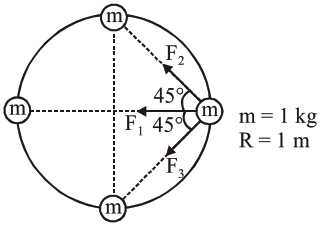# Four identical particles of

Question:

Four identical particles of equal masses $1 \mathrm{~kg}$ made to move along the circumference of a circle of radius $1 \mathrm{~m}$ under the action of their own mutual gravitational attraction. The speed of each particle will be :

1. $\sqrt{\frac{G}{2}(1+2 \sqrt{2})}$

2. $\sqrt{G(1+2 \sqrt{2})}$

3. $\sqrt{\frac{G}{2}(2 \sqrt{2}-1)}$

4. $\sqrt{\frac{(1+2 \sqrt{2}) G}{2}}$

Correct Option: , 4

Solution:$\mathrm{F}_{1}=\frac{\mathrm{Gmm}}{(2 \mathrm{R})^{2}}=\frac{\mathrm{Gm}^{2}}{4 \mathrm{R}^{2}}$

$\mathrm{~F}_{2}=\frac{\mathrm{Gmm}}{(\sqrt{2} \mathrm{R})^{2}}=\frac{\mathrm{Gm}^{2}}{2 \mathrm{R}^{2}}$

$\mathrm{~F}_{3}=\frac{\mathrm{Gmm}}{(\sqrt{2} \mathrm{R})^{2}}=\frac{\mathrm{Gm}^{2}}{2 \mathrm{R}^{2}}$

$\Rightarrow \mathrm{F}_{\text {net }}=\mathrm{F}_{1}+\mathrm{F}_{2} \cos 45^{\circ}+\mathrm{F}_{3} \cos 45^{\circ}$

$=\frac{\mathrm{Gm}^{2}}{4 \mathrm{R}^{2}}+\frac{\mathrm{Gm}^{2}}{2 \mathrm{R}^{2}} \frac{1}{\sqrt{2}}+\frac{\mathrm{Gm}^{2}}{2 \mathrm{R}^{2}} \frac{1}{\sqrt{2}}$

$=\frac{\mathrm{Gm}^{2}}{\mathrm{R}^{2}}\left(\frac{1}{4}+\frac{1}{2 \sqrt{2}}+\frac{1}{2 \sqrt{2}}\right)$

$=\frac{\mathrm{Gm}^{2}}{\mathrm{R}^{2}}\left(\frac{1}{4}+\frac{1}{\sqrt{2}}\right)=\frac{\mathrm{Gm}^{2}}{4 \mathrm{R}^{2}}(1+2 \sqrt{2})$

$\mathrm{F}_{\mathrm{net}}=\frac{\mathrm{Gm}^{2}}{4 \mathrm{R}^{2}}(1+2 \sqrt{2})=\frac{\mathrm{mv}^{2}}{\mathrm{R}}$

$\Rightarrow \mathrm{v}=\frac{\sqrt{\mathrm{G}(1+2 \sqrt{2})}}{2}$